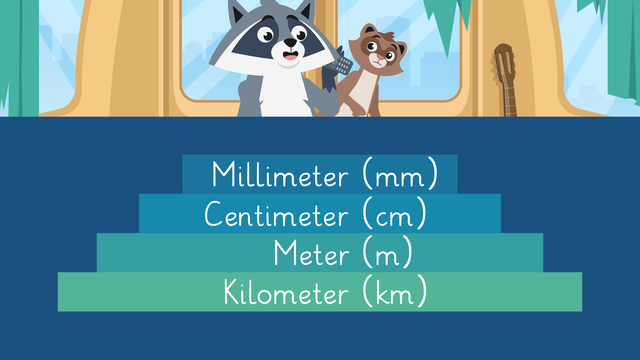# Millimeters, Centimeters, Meters and KilometersRating

Ø 5.0 / 1 ratings

The authorsTeam Digital
Millimeters, Centimeters, Meters and Kilometers
CCSS.MATH.CONTENT.4.MD.A.1

## Basics on the topicMillimeters, Centimeters, Meters and Kilometers

### In This Video on Kilometers, Meters, Centimeters, and Millimeters

Freddie orders a measuring kit after seeing an infomercial on TV. They learn all about meters and kilometers, and also centimeters and millimeters! After this video, you will be able to explain what centimeters, kilometers, and millimeters are, and be able to tell the difference between them.

### What are Centimeters, Kilometers, and Millimeters?

What is the relationship between centimeters, millimeters, meters, and kilometers? They are all metric measurements of length! From smallest to largest measurement, we have millimeters, centimeters, meters, and kilometers!Look at the above chart. What is between millimeters and kilometers? Centimeters and meters are between millimeters and kilometers!

### Metric Measurement Relationship

How do you relate meters (m), centimeters (cm), millimeters (mm) and kilometers (km)? Each unit of measurement relates to the others, because you have so many of one, in a number of the other.

For example, let’s look at millimeters first. There is one million millimeters in a kilometer, one thousand millimeters in one meter, and one hundred millimeters in a centimeter.Now let’s look at centimeters. There is one hundred thousand centimeters in a kilometer, and one hundred centimeters in a meter.Now let’s look at meters. There is one thousand meters in a kilometer.### How Big is Millimeters, Centimeters, Kilometers, and Meters?

A millimeter is roughly the size of the thickness of a paper clip.A centimeter is roughly the length of a bug.A meter is roughly the length of a guitar.A kilometer is roughly the distance between the support towers on the Golden Gate Bridge.### Kilometers and Meters and Millimeters and Centimeters - Summary

Millimeters, centimeters, meters, and kilometers are all metric units of measurement.They are related because you can convert one to the other! Below you will find a worksheet for extra practice.

### TranscriptMillimeters, Centimeters, Meters and Kilometers

"If you call right now, you will get TWO measuring kits for the price of ONE, delivered immediately!" "I can't believe people fall for that, can you Zuri?" "I'll take two measuring kits, please!" "Well, you've purchased it now, might as well take a look." Let's join Zuri and Freddie as they learn all about millimeters, centimeters, Meters, and kilometers. Metric measurements, or units, of length that we use are kilometers, meters, centimeters, and millimeters. A millimeter is a small measure of length, and is represented by the abbreviation mm. A centimeter is a bigger measurement than millimeters, and is represented by the abbreviation cm. A meter is a bigger measurement than a centimeter, and is represented by the abbreviation m. A kilometer is a bigger measurement than a meter, and is represented by the abbreviation km. Let's take a closer look at millimeters! Ten millimeters make up one centimeter, one thousand millimeters make up one meter, and one million millimeters make up one kilometer. In fact the word milli in millimeter means million. You can use this to remember that a million millimeters make up one kilometer. Now let's take a look at centimeters! One hundred centimeters make up one meter, and one hundred thousand centimeters make up one kilometer. In fact, the word centi in centimeter is from the word century, which means one hundred. You can use this to remember that one hundred centimeters make up one meter. Now let's take a look at meters! One thousand meters make up one kilometer. And finally, let's look at kilometers! This is the biggest unit of length we are seeing here, and we can remember how many meters make up one kilometer by looking at the word kilo. Kilo means one thousand. You can use this to remember that a thousand meters make up one kilometer. What are some items or objects that are about the size of each unit? The thickness of a paperclip is roughly one millimeter. Some insects can be roughly one centimeter long. A guitar is roughly one meter. For one kilometer, there isn't a specific item that is one kilometer long, but the distance between the support towers on the Golden Gate Bridge is pretty close at just over one kilometer! While Zuri and Freddie finish up exploring the measuring kit, let's review! Remember, a millimeter is a small measure of length, and is represented by the abbreviation mm. A centimeter is a bigger measurement than millimeters, and is represented by the abbreviation cm. A meter is a bigger measurement than a centimeter, and is represented by the abbreviation mm. A kilometer is a bigger measurement than a meter, and is represented by the abbreviation km. "Order now to get my Weighted Wonders kit!" "No Zuri, Please don't." "I'll take one of the Weighted Wonders kits please!"